## MP Board Class 6th Maths Solutions Chapter 3 Playing With Numbers Ex 3.5

Question 1.
Which of the following statements are true?
(a) If a number is divisible by 3, it must be divisible by 9.
(b) If a number is divisible by 9, it must be divisible by 3.
(c) A number is divisible by 18, if it is divisible by both 3 and 6.
(d) If a number is divisible by 9 and 10 both, then it must be divisible by 90.
(e) If two numbers are co-primes, at least one of them must be prime.
(f) All numbers which are divisible by 4 must also be divisible by 8.
(g) All numbers which are divisible by 8 must also be divisible by 4.
(h) If a number exactly divides two numbers separately, it must exactly divide their sum.
(i) If a number exactly divides the sum of two numbers, it must exactly divide the two numbers separately.
Solution:
Statements (b), (d), (g) and (h) are true.Question 2.
Here are two different factor trees for 60. Write the missing numbers.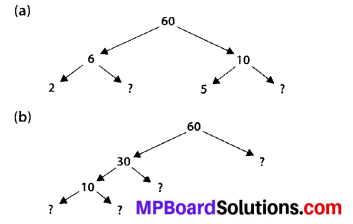Solution:
Factors of 60 are 1, 2, 3, 4, 5, 6,10,12,15, 20, 30, 60.
(a) Since 6 = 2 × 3 and 10 = 5 × 2
∴ The missing numbers are 3 and 2.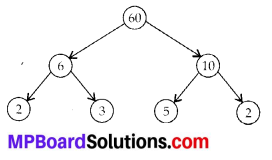(b) Since, 60 = 30 × 2, 30 = 10 × 3, and 10 = 5 × 2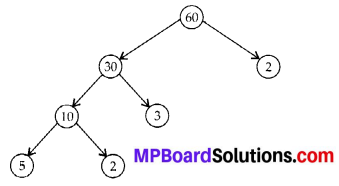Question 4.
Write the greatest 4-digit number and express it in terms of its prime factors.
Solution:
The greatest four digit number is 9999.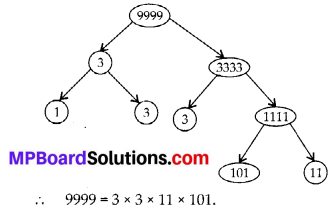Question 5.
Write the smallest 5-digit number and express it in the form of its prime factors.
Solution:
The smallest five digit number is 10000.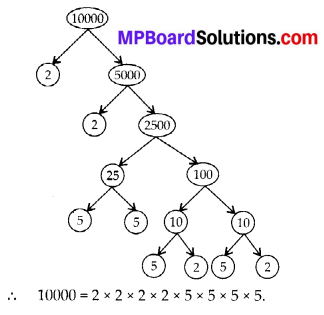Question 6.
Find all the prime factors of 1729 and arrange them in ascending order. Now state the relation, if any; between two consecutive prime factors.
Solution: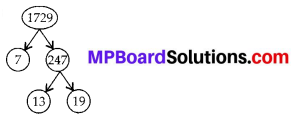∴ 1729 = 7 × 13 × 19.
The difference of two consecutive prime factors is 6. (∵ 13 – 7 = 6 and 19 – 13 = 6)Question 7.
The product of three consecutive numbers is always divisible by 6. Verify this statement with the help of some examples.
Solution:
Among the three consecutive numbers, there must be atleast one even number and one multiple of 3. Thus, the product must be divisible by 6.
For example :
(i) 2 × 3 × 4 = 24
(ii) 4 × 5 × 6 = 120,
where both 24 and 120 are divisible by 6.Question 8.
The sum of two consecutive odd numbers is divisible by 4. Verify this statement with the help of some examples.
Solution:
The sum of two consecutive odd numbers is divisible by 4.
For example : 3 + 5 = 8 and 8 is divisible by 4.
5 + 7 = 12 and 12 is divisible by 4.
7 + 9 = 16 and 16 is divisible by 4.
9 + 11 = 20 and 20 is divisible by 4.

Question 9.
In which of the following expressions, prime factorisation has been done?
(a) 24 = 2 × 3 × 4
(b) 56 = 7 × 2 × 2 × 2
(c) 70 = 2 × 5 × 7
(d) 54 = 2 × 3 × 9
Solution:
In expressions (b) and (c), prime factorisation has been done.Question 10.
Determine if 25110 is divisible by 45.
[Hint: 5 and 9 are co-prime numbers. Test the divisibility of the number by 5 and 9],
Solution:
The prime factorisation of 45 = 5 × 9
25110 is divisible by 5 as ‘0’ is at its unit place.
25110 is divisible by 9 as sum of digits (i.e., 9) is divisible by 9.
Therefore, the number 25110 must be divisible by 5 × 9 = 45

Question 11.
18 is divisible by both 2 and 3. It is also divisible by 2 × 3 = 6. Similarly, a number is divisible by both 4 and 6. Can we say that the number must also be divisible by 4 × 6 = 24? If not, give an example to justify your answer.
Solution:
No. The number 12 is divisible by both 6 and 4, but 12 is not divisible by 24.
∴ A number divisible by both 4 and ( may or may not be divisible by 4 × 6 = 24.Question 12.
I am the smallest number, having four different prime factors. Can you find me?
Solution:
Since, 2 × 3 × 5 × 7 = 210
∴ 210 is the smallest number, having 4
different prime factors i.e., 2, 3, 5 and 7.